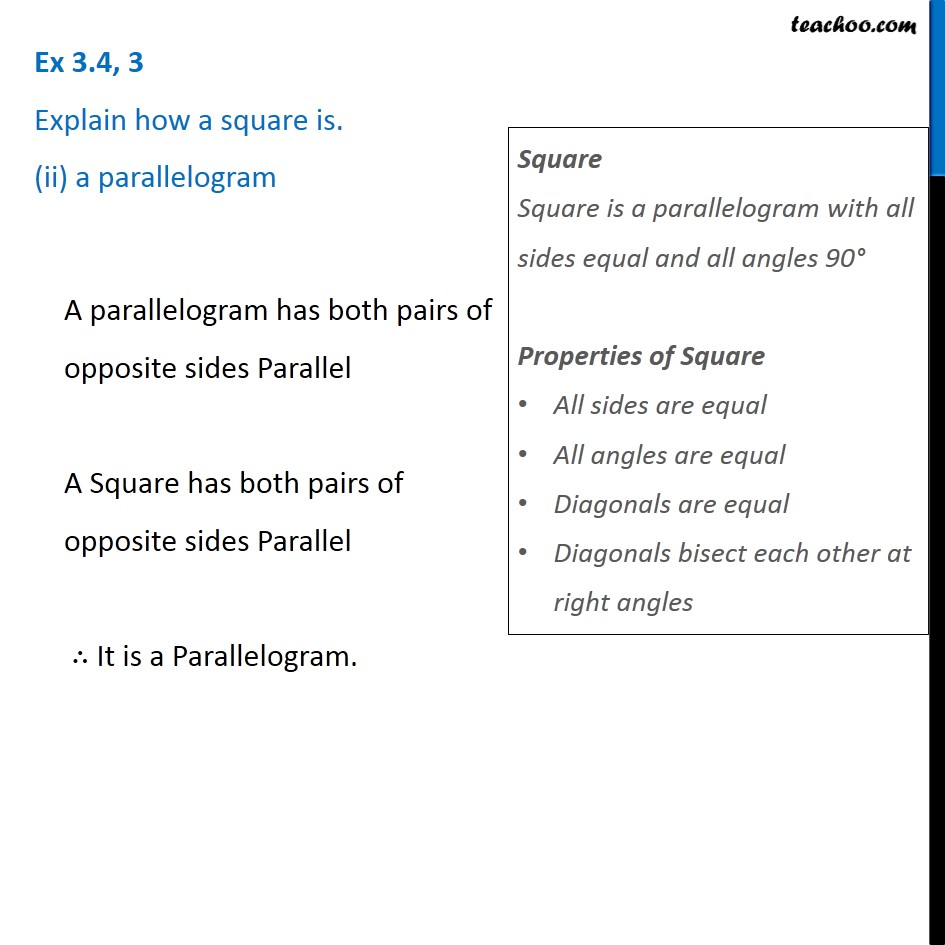Ex 3.4

Chapter 3 Class 8 Understanding Quadrilaterals
Serial order wiseLearn in your speed, with individual attention - Teachoo Maths 1-on-1 Class

### Transcript

Ex 3.4, 3 Explain how a square is. (ii) a parallelogram A parallelogram has both pairs of opposite sides Parallel A Square has both pairs of opposite sides Parallel ∴ It is a Parallelogram. Square Square is a parallelogram with all sides equal and all angles 90° Properties of Square All sides are equal All angles are equal Diagonals are equal Diagonals bisect each other at right angles